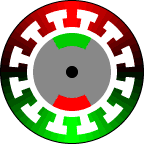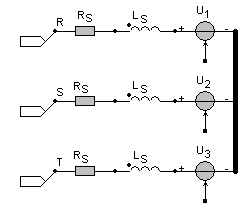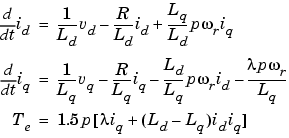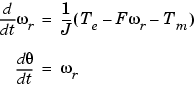• Introduction
• What is in this manual
• What is Caspoc
• User interface
• Introduction
• Starting
• Simulation
• Editing
• Viewing and printing
• Getting Started
• Basic editing
• Simulation in the time domain
• Basic User Interface Topics
• Editing
• Simulation
• Viewing
• Library
• Reports
• Project management
• Circuit and Block Diagram Components
• Introduction
• Cscript and user defined functions
• Component parameters
• Modeling Topics
• Introduction
• Power Electronics
• Semiconductors
• Electrical Machines
• Electrical drives
• Power Systems
• Mechanical Systems
• Thermal Systems
• Magnetic Circuits
• Green Energy
• Coupling to FEM
• Experimenter
• Analog hardware description language
• Embedded C code Export
• Coupling to Spice
• Small Signal Analysis
• Matlab coupling
• Tips and tricks
• Appendices

## Permanent Magnet Synchronous MachinesClick to Close this View Usually, a brushless dc motor is commutated. One stator pole is turned-off and the next pole is energized. The windings of a brushless dc motor are distinct separate poles. This arrangement is the most simple to wind and control, but produces some torque ripple.

A variation of a brushless dc motor uses a slightly different winding. The windings are distributed around the stator to produce a roughly sinusoidal distribution. The stator winding is similar to an ac induction motor. This type of motor is called a brushless ac motor or Permanent Magnet Synchronous Machine (PMSM).

A PMSM is driven with ac sinewave voltages. The permanent magnet rotor rotates synchronous to the rotating magnetic field. The rotating magnetic field is illustrated using a red and green gradient. An actual simulation of the magnetic field would show a far more complex magnetic field.

The basic block BLDCM from the block diagram models the Permanent Magnet Synchronous Machine. The output equals the electric torque produced by the machine. The inputs are the three phase currents, the angular speed of the rotor and the machine constant for the calculation of the torque and the produced EMF.

u1:=K*sin(angle);u2:=K*sin(angle-(2*PI/3));u3:=K*sin(angle-(4*PI/3));if(p1=0)then  begin  if(u1 < 0) then u1:=K else u1:=-K;  if(u2 < 0) then u2:=K else u2:=-K;  if(u3 < 0) then u3:=K else u3:=-K;  end;T=u1*i1+u2*i2+u3*i3

Inside the basic BLDCM block from the block diagram the type of the machine can be defined.

p1=0 Brushless DC machine BLDCM
p1=1 Permanent Magnet Synchronous Machine

Use an INT_VAR block to get the EMF voltages and position of the rotor:

 INT_VAR Output p1=1 u1 p1=2 u2 p1=3 u3 p1=4 Position of the rotor (in Radians) p1=5 Rotor position polar x-coordinate p1=6 Rotor position polar y-coordinate

Equivalent circuit model

The library blocks for the Permanent Magnet Synchronous Machine have electrical and mechanical connections. The electrical part of the Permanent Magnet Synchronous Machine is modeled by a series connection of the stator inductance, resistance and the above mentioned EMF.The connections R, S and T are the electrical terminals. The stator current is depends on the produced EMF:

us=Rs · is+Lsdis/dt+uEMF)

where uEMFr*ui

The torque produced by the machine equals:

Te=u1 · i1+u2 · i2+u3 · i3

Mechanical part:

The mechanical shaft is modeled by a rigid shaft model. The inertia J [kgm2]of the shaft can be modeled in most of the Permanent Magnet Synchronous Machine library blocks. Most machine models have internal parameters for modeling the inertia and friction of the shaft. The Permanent Magnet Synchronous Machine can either run without load or can be connected to a mechanical rotational model. Use the models from components/circuit/rotational and from the various mechanical libraries for modeling the mechanical model.

DQ-model

There are also models for the Permanent Magnet Synchronous Machine in the library that are based on the dq model. The dq model for the PMSM models the dynamics of a three-phase permanent magnet synchronous machine with sinusoidal flux distribution.

The electrical and mechanical parts of the machine are each represented by a second-order state-space model. The model assumes that the flux established by the permanent magnets in the stator is sinusoidal, which implies that the electromotive forces are sinusoidal.

The block implements the following equations expressed in the rotor reference frame (qd frame).

Electrical Systemwhere (all quantities in the rotor reference frame are referred to the stator)

 Lq, Ld q and d axis inductance's R Resistance of the stator windings iq, id q and d axis currents vq, vd q and d axis voltages ωr Angular velocity of the rotor λ Amplitude of the flux induced by the permanent magnets of the rotor in the stator phases p Number of pole pairs Te Electromagnetic torque

Mechanical Systemwhere

 J Rotor inertia F Rotor friction θ Rotor angular position Tm Shaft mechanical torque

With excitation winding

Here the parameter Lm defines the coupling between Ld and the exitation winding Le

Ψd=Ld*id+Lm*ie
Ψe=Lm*id+Le*ie
 Le Excitation winding inductance Re Excitation winding resistance Lm Mutual inductance between the excitation winding and Ld